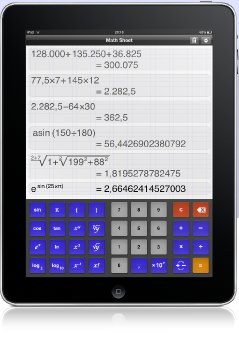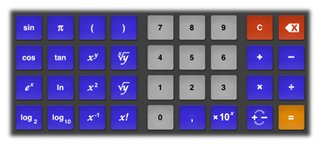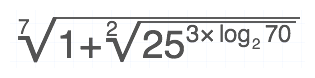# Math Sheet for iPad

The Calculator you have ever wanted# Introducing Math Sheet

Math Sheet is the first calculator following the design philosphy of the iPad. In contrast to other calculators on the App Store, Math Sheet does not imitate primitive electronics. Instead, it provides a virtual sheet of paper for writing down math problems. You always see what you typed, allowing you to detect and instantly correct typos. The result of each calculation is depicted on the virtual paper along with the history of previous calculations. Thus, you do not need a separate sheet of paper only for keeping intermediate results.

Simplicity and user friendliness were our core design goals. As a result, Math Sheet is very simple to use even for the casual user. Correct and precise calculation with support for advanced functions make this calculator convenient for scientific work.

# The math keyboardMath Sheet provides an optimized keyboard for entering calculations. The keyboard is of similar size as the built-in virtual keyboard of the iPad in order to allow fast and error free input. Most functions are available on the default keyboard. However, some additional functions can only be reached by accessing the second function of a key. The second function is available by pressing the key twice. When you press the key for the first time, the key changes its color and title. This method of input allows much faster typing than providing a „2nd” key for accessing the additional function.

# The rendererMath sheet includes a sophisticated renderer for mathematical equations. It supports complex formulas and renders a quality comparable to what you find in a math book. Readability and ease of use were the drivers behind the design of the renderer. It therefore adds parenthesis to structure the input while keying in an equation. As soon as the input is complete, it omits the superfluous parenthesis to reduce visual distraction.

# The math engine

Math sheet provides all common functions you would expect from a scientific calculator. It offers a precision of 15 digits and supports the value range of -10-99 to 1099. The following functions are available in the standard version:

• Basic math: addition, subtraction, multiplication, division
• Unlimited levels of parenthesis
• Power: square, cube, reciprocal, power
• Roots: square root, cube root, nth root
• Trigonometric functions: sinus, cosinus, tangens, arcus sinus, arcus cosinus, arcus tangens
• Constants: e, π
• Logarithms: logarithmus dualis, logrithmus naturalis, logarithmus decimalis
• Exponential function: ex
• Factorial/Gamma function

# Feedback

Please give us feedback on how you use Math Sheet and which functions you would like to see in future versions. An iPhone version with an adapted user interface for the smaller display and extensions that make the application even more useful will follow soon.

Feedback can be given by sending an e-mail to support@math-sheet.de.